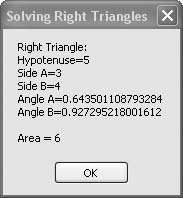# Recipe 6.17. Solving Right Triangles

#### Problem

You want to calculate all the remaining sides and angles of a right triangle given two known parts of the triangle.

#### Solution

Sample code folder: Chapter 06\RightTriangle

Create a RightTriangle class that calculates all parts of a right triangle given any two of its parts.

#### Discussion

The parts of a right triangle we are concerned with are the two sides A and B adjacent to the right angle, the hypotenuse (the side opposite the right angle), and the two angles formed where the hypotenuse meets sides A and B. If you know any two of these values, all the rest can be determined.

There are many ways to set up the RightTriangle class, and the technique chosen here is not the only reasonable approach to the problem. We chose to use the initializing function New() to define the triangle by passing in nonzero numbers for the known parts and a value of zero for the unknowns. The IntelliSense pop-up prompt makes it easy to remember what parts of the triangle are passed in at each parameter position. It's as easy as filling in the blanks. The code for the RightTriangle class is as follows:

` Public Class RightTriangle    Private StoredSideA As Double    Private StoredSideB As Double    Private StoredHypotenuse As Double    Private StoredAngleA As Double    Private StoredAngleB As Double    Public Sub New(ByVal hypotenuse As Double, _          ByVal sideA As Double, ByVal sideB As Double, _          ByVal angleA As Double, ByVal angleB As Double)       Me.StoredHypotenuse = hypotenuse       Me.StoredSideA = sideA       Me.StoredSideB = sideB       Me.StoredAngleA = angleA       Me.StoredAngleB = angleB       Me.Resolve()    End Sub    Public ReadOnly Property SideA() As Double       Get          Return StoredSideA       End Get    End Property    Public ReadOnly Property SideB() As Double       Get          Return StoredSideB       End Get    End Property    Public ReadOnly Property AngleA() As Double       Get          Return StoredAngleA       End Get    End Property    Public ReadOnly Property AngleB() As Double       Get          Return StoredAngleB       End Get    End Property    Public ReadOnly Property Hypotenuse() As Double       Get          Return StoredHypotenuse       End Get    End Property    Private Sub Resolve()       ' ----- Figure out the missing (zero) parts of the       '       triangle. Start with the angles.       If (StoredAngleA = 0.0#) And _          (StoredAngleB <> 0.0#) Then _          StoredAngleA = Math.PI / 2 - StoredAngleB       If (StoredAngleB = 0.0#) And _          (StoredAngleA <> 0.0#) Then _          StoredAngleB = Math.PI / 2 - StoredAngleA       If (StoredAngleA <> 0.0#) And _          (StoredHypotenuse <> 0.0#) Then _          StoredSideB = StoredHypotenuse * _          Math.Cos(StoredAngleA)       If (StoredAngleB <> 0.0#) And _          (StoredHypotenuse <> 0.0#) Then _          StoredSideA = StoredHypotenuse * _          Math.Cos(StoredAngleB)       If (StoredAngleA <> 0.0#) And _          (StoredSideA <> 0.0#) Then _          StoredHypotenuse = StoredSideA / _          Math.Sin(StoredAngleA)       If (StoredAngleB <> 0.0#) And _          (StoredSideB <> 0.0#) Then _          StoredHypotenuse = StoredSideB / _          Math.Sin(StoredAngleB)       If (StoredAngleA <> 0.0#) And _          (StoredSideB <> 0.0#) Then _          StoredHypotenuse = StoredSideB / _          Math.Cos(StoredAngleA)       If (StoredAngleB <> 0.0#) And _          (StoredSideA <> 0.0#) Then _          StoredHypotenuse = StoredSideA / _          Math.Cos(StoredAngleB)       ' ----- Now calculate the sides.       If (StoredSideA <> 0.0#) And _          (StoredSideB <> 0.0#) Then _          StoredHypotenuse = Math.Sqrt(StoredSideA ^ 2 + _          StoredSideB ^ 2)       If (StoredSideA <> 0.0#) And _          (StoredHypotenuse <> 0.0#) Then _          StoredSideB = Math.Sqrt(StoredHypotenuse ^ 2 - _          StoredSideA ^ 2)       If (StoredSideB <> 0.0#) And _          (StoredHypotenuse <> 0.0#) Then _          StoredSideA = Math.Sqrt(StoredHypotenuse ^ 2 - _          StoredSideB ^ 2)       If (StoredAngleA = 0.0#) Then StoredAngleA = _          Math.Asin(StoredSideA / StoredHypotenuse)       If (StoredAngleB = 0.0#) Then StoredAngleB = _          Math.Asin(StoredSideB / StoredHypotenuse)    End Sub    Public Overrides Function Tostring() As String       ' ----- Display all values of the triangle.       Dim result As New System.Text.StringBuilder       result.AppendLine("  Right Triangle:")       result.AppendLine("Hypotenuse=" & _           StoredHypotenuse.ToString)       result.AppendLine("Side A=" & StoredSideA.ToString)       result.AppendLine("Side B=" & StoredSideB.ToString)       result.AppendLine("Angle A=" & StoredAngleA.ToString)       result.Append("Angle B=" & StoredAngleB.ToString)       Return result.ToString()    End Function End Class `

The core calculations of this class are performed in the private Resolve() function. There, the various triangle parts are tested to see if they are nonzero, and the appropriate calculations are performed to start filling in the blanks for the unknowns. Resolve() is called just once, at the moment when the RightTriangle object is instantiated. All the parts of the right triangle are later returned as required via read-only properties.

Visual Basic internally always assumes angles to be in radians, even though degrees are the most commonly used units for angles among the general population. It's tempting to use degrees in user-defined classes and procedures, but for consistency this book will assume radians throughout.

The following sample code creates an instance of the RightTriangle object and uses it to calculate a typical right triangle. In this example, the lengths of sides A and B are known. All other parts of the triangle are passed as zero when the RightTriangle is instantiated:

` Dim testTriangle As RightTriangle Dim area As Double testTriangle = New RightTriangle(0, 3, 4, 0, 0) area = (testTriangle.SideA * testTriangle.SideB) / 2 MsgBox(testTriangle.Tostring & vbNewLine & _    "Area = " & area.ToString) `

Figure 6-17 shows the results of calculating the missing parts of a right triangle with sides A and B of lengths 3 and 4.

##### Figure 6-17. Using the RightTriangle class to calculate unknown parts of a right triangleVisual Basic 2005 Cookbook: Solutions for VB 2005 Programmers (Cookbooks (OReilly))
ISBN: 0596101775
EAN: 2147483647
Year: 2006
Pages: 400

Similar book on Amazon